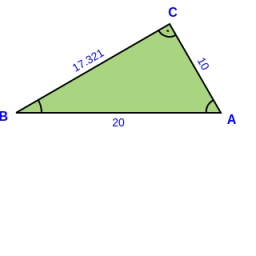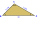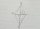# Acute triangle

In the acute triangle KLM, V is the intersection of its heights and X is the heel of height to the side KL. The axis of the angle XVL is parallel to the side LM and the angle MKL is 70°. What size are the KLM and KML angles?

KLM =  55 °
KML =  55 °

### Step-by-step explanation:

$KML=180-MKL-KLM=180-70-55=55\text{°}$Did you find an error or inaccuracy? Feel free to write us. Thank you!Tips to related online calculators

#### You need to know the following knowledge to solve this word math problem:

We encourage you to watch this tutorial video on this math problem:

## Related math problems and questions:

• Inner anglesThe magnitude of the internal angle at the main vertex C of the isosceles triangle ABC is 72°. The line p, parallel to the base of this triangle, divides the triangle into a trapezoid and a smaller triangle. How big are the inner angles of the trapezoid?
• MO Z7–I–6 2021In the triangle ABC, point D lies on the AC side and point E on the BC side. The sizes of the angles ABD, BAE, CAE and CBD are 30°, 60°, 20° and 30°, respectively. Find the size of the AED angle.
• Internal anglesThe ABCD is an isosceles trapezoid, which holds: |AB| = 2 |BC| = 2 |CD| = 2 |DA|: On its side BC is a K point such that |BK| = 2 |KC|, on its side CD is the point L such that |CL| = 2 |LD|, and on its side DA the point M is such that | DM | = 2 |MA|. Dete
• Outer anglesThe outer angle of the triangle ABC at the A vertex is 71°40 ' outer angle at the vertex B is 136°50'. What size has the inner triangle angle at the vertex C?
• KLM triangleFind the length of the sides of the triangle KLM if m = 5cm height to m = 4.5 cm and size MKL angle is 70 degrees.
• TrianglesFind out whether given sizes of the angles can be interior angles of a triangle: a) 23°10',84°30',72°20' b) 90°,41°33',48°37' c) 14°51',90°,75°49' d) 58°58',59°59',60°3'
• SimilarityAre two right triangles similar to each other if the first one has an acute angle 70°, and the second one has an acute angle 20°?
• The twoThe two angles of a triangle are 78° and 82°. So what is the measure of the remaining third angle?
• Right angleIn a right triangle ABC with a right angle at the apex C, we know the side length AB = 24 cm and the angle at the vertex B = 71°. Calculate the length of the legs of the triangle.
• Angles in ratioThe size of the angles of the triangle are in ratio x: y = 7: 5 and the angle z is 42° lower than the angle y. Find size of the angles x, y, z.
• In a 2In a thirteen sided polygon, the sum of five angles is 1274°, four of the eight angles remaining are equal and the other four are 18° less than each of the equal angles. Find the angles. .
• Angles in triangleThe triangle is ratio of the angles β:γ = 6:8. Angle α is 40° greater than β. What are the size of angles of the triangle?
• AngleA straight line p given by the equation y = (-8)/(6) x +78. Calculate the size of angle in degrees between line p and y-axis.
• Diamond and anglesFind the area of a diamond with a side of 5 cm if you know that the internal angles in the diamond are 60° and 120°.
• Powerplant chimneyFrom the building window at the height of 7.5 m, we can see the top of the factory chimney at an altitude angle of 76° 30 ′. We can see the chimney base from the same place at a depth angle of 5° 50 ′. How tall is the chimney?
• A kiteABCD is a kite. Angle OBC = 20° and angle OCD = 35°. O is the intersection of diagonals. Find angle ABC, angle ADC and angle BAD.The ladder touch on a wall at a height of 7.5 m. The angle of the inclination of the ladder is 76°. How far is the lower end of the ladder from the wall?